# Extinction and decay estimates of solutions for a porous medium equation with nonlocal source and strong absorption

## Abstract

In this paper, we investigate extinction properties of the solutions for the initial Dirichlet boundary value problem of a porous medium equation with nonlocal source and strong absorption terms. We obtain some sufficient conditions for the extinction of nonnegative nontrivial weak solutions and the corresponding decay estimates which depend on the initial data, coefficients, and domains.

## 1 Introduction

We consider the initial Dirichlet boundary value problem for a class of porous medium equations with nonlocal source and strong absorption terms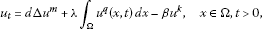(1)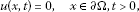(2)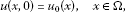(3)

where $0, $d,\lambda ,\beta ,q>0$, $\mathrm{\Omega }\subset {R}^{N}$ ($N\ge 1$) is a bounded domain with smooth boundary, and ${u}_{0}\left(x\right)\in {L}^{\mathrm{\infty }}\left(\mathrm{\Omega }\right)\cap {W}_{0}^{1,2}\left(\mathrm{\Omega }\right)$ is a nonnegative function. The symbols ${\parallel \cdot \parallel }_{p}$ and ${\parallel \cdot \parallel }_{1,p}$, where $p\ge 1$, denote ${L}^{p}\left(\mathrm{\Omega }\right)$- and ${W}^{1,p}\left(\mathrm{\Omega }\right)$-norm, respectively, and $|\mathrm{\Omega }|$ denotes the measure of Ω.

Equation (1) describes the fast diffusion of concentration of some Newtonian fluids through a porous medium or the density of some biological species in many physical phenomena and biological species theories, while nonlocal source and absorption terms cooperate and interact with each other during the diffusion. It has been known that the nonlocal source term presents a more realistic model for population dynamics; see . In the nonlinear diffusion theory, obvious differences exist among the situations of slow ($m>1$), fast ($0), and linear ($m=1$) diffusions. For example, there is a finite speed propagation in the slow and linear diffusion situations, whereas an infinite speed propagation exists in the fast diffusion situation.

Recently, many scholars have been devoted to the study of blow-up and extinction properties of solutions for nonlinear parabolic equations with nonlocal terms. The blow-up rates and blow-up sets of solutions to equation (1) have been investigated when $d=\lambda =1$, $m\ge 1$, and the linear absorption term is replaced with a nonlinear term with exponent (cf. ). Extinction is the phenomenon whereby there exists a finite time $T>0$ such that the solution is nontrivial for $0 and then $u\left(x,t\right)\equiv 0$ for all $\left(x,t\right)\in \mathrm{\Omega }×\left[T,+\mathrm{\infty }\right)$. In this case, T is called the extinction time. It is also an important property of solutions for nonlinear parabolic equations which have been studied by many researchers. For instance, Evans and Knerr  investigated the extinction behaviors of solutions for the Cauchy problem of the semilinear parabolic equation

${u}_{t}\left(x,t\right)=\mathrm{\Delta }u\left(x,t\right)-\beta \left(u\left(x,t\right)\right),\phantom{\rule{1em}{0ex}}x\in {R}^{n},t>0,$

by constructing a suitable comparison function. Ferreira and Vazquez  studied the extinction phenomena of solutions for the Cauchy problem of the porous medium equation with an absorption term

${u}_{t}={\left({u}^{m}\right)}_{xx}-{u}^{p},\phantom{\rule{1em}{0ex}}x\in R,t>0,$

by using the analysis of self-similar solutions. Li and Wu  considered the problem of the porous medium equation with a source term

${u}_{t}=\mathrm{\Delta }{u}^{m}+\lambda {u}^{p},\phantom{\rule{1em}{0ex}}x\in \mathrm{\Omega },t>0,$
(4)

subject to (2) and (3). They obtained some conditions for the extinction and non-extinction of solutions to equation (4) and decay estimates by the upper and lower solutions method. On extinctions of solutions to the p-Laplacian equations or the doubly degenerate equations, we refer readers to [13, 14] and the references therein.

Replacing the nonlocal term in equation (1) with a local term, Liu et al. considered the initial Dirichlet boundary value problem for a class of porous medium equations

${u}_{t}=\mathrm{\Delta }{u}^{m}+{u}^{q}-\beta u,\phantom{\rule{1em}{0ex}}x\in \mathrm{\Omega },t>0,$

and obtained sufficient conditions for the extinction and non-extinction of solutions to that equation. Thereafter, Fang and Li  extended their results to the doubly degenerate equation in the whole dimensional space.

For equation (1) with $\beta =0$, $q>0$, and $N>2$, Han and Gao  showed that $q=m$ is the critical exponent for the occurrence of extinction or non-extinction. When $m=1$, $q>0$, and $N>2$, the conditions for the extinction and non-extinction of solutions and corresponding decay estimates were obtained (cf. ). Recently, Fang and Xu  considered equation (1) with $k=1$, when the diffusion term was replaced with a p-Laplacian operator in the whole dimensional space, and showed that the extinction of the weak solution is determined by the competition of two nonlinear terms. They also obtained the exponential decay estimates which depend on the initial data, coefficients, and domains. The extinctions of solutions to equation (1) with nonlocal source terms do not depend on the first eigenvalue of the corresponding operator, which is different from the case of local source terms. The extinction and decay estimates for solutions to the nonlocal fast diffusion equations with nonzero coefficients and strong absorption terms, like equation (1), are still being investigated.

Motivated by the above works, we investigate whether the existence of strong absorption can change extinction behaviors for solutions to problem (1)-(3) in the whole dimensional space. The main tools we use are the integral estimate method and the Gagliardo-Nirenberg inequality. This technique has a wide application, especially for equations that do not satisfy the maximum principle (cf. ). Our goals are to show that the extinction of nonnegative nontrivial weak solutions to problem (1)-(3) occurs when $0 and to find the decay estimates depending on the initial data, coefficients, and domains.

Our paper is organized as follows. In Section 2, we give preliminary knowledge including lemmas that are required in the proofs of our results and present the proofs for the results in Section 3.

## 2 Preliminary knowledge

Due to the singularity of equation (1), problem (1)-(3) has no classical solutions in general. To state the definition of the weak solution, we let ${Q}_{T}=\mathrm{\Omega }×\left(0,T\right)$ and firstly define the class of nonnegative testing functions

$F=\left\{\xi :\xi \in C\left({\overline{Q}}_{T}\right)\cap {C}^{2,1}\left({Q}_{T}\right),{\xi }_{t},\mathrm{\Delta }\xi \in {L}^{2}\left({Q}_{T}\right);\xi \ge 0,\xi {|}_{\partial \mathrm{\Omega }×\left(0,T\right)}=0\right\}.$

Definition 1 A function $u\in {L}^{\mathrm{\infty }}\left({Q}_{T}\right)$ is called a weak subsolution (supersolution) of problem (1)-(3) in ${Q}_{T}$ if the following conditions hold:

1. a.

$u\left(x,0\right)\le \left(\ge \right)\phantom{\rule{0.2em}{0ex}}{u}_{0}\left(x\right)$ in Ω;

2. b.

$u\left(x,t\right)\le \left(\ge \right)\phantom{\rule{0.2em}{0ex}}0$ on $\partial \mathrm{\Omega }×\left(0,T\right)$;

3. c.

For every $t\in \left(0,T\right)$ and every $\xi \in F$,

$\begin{array}{rcl}{\int }_{\mathrm{\Omega }}u\left(x,t\right)\xi \left(x,t\right)\phantom{\rule{0.2em}{0ex}}dx& \le & {\int }_{\mathrm{\Omega }}{u}_{0}\left(x\right)\xi \left(x,0\right)\phantom{\rule{0.2em}{0ex}}dx+{\int }_{0}^{T}{\int }_{\mathrm{\Omega }}\left\{u{\xi }_{s}+d{u}^{m}\mathrm{\Delta }\xi \\ +\lambda {\int }_{\mathrm{\Omega }}{u}^{q}\left(y,s\right)\phantom{\rule{0.2em}{0ex}}dy\xi \left(x,s\right)-\beta {u}^{k}\left(x,s\right)\xi \left(x,s\right)\right\}\phantom{\rule{0.2em}{0ex}}dx\phantom{\rule{0.2em}{0ex}}ds.\end{array}$

A function u is called a locally weak solution of problem (1)-(3) if it is both a subsolution and a supersolution for some $T>0$.

Remark 1 The existence and uniqueness of locally nonnegative solutions in time to problem (1)-(3) can be obtained by the standard parabolic regular theory that can be applied to get suitable estimates in the standard limiting process (cf. [2, 21, 22]). The proof is similar to the ones in the cited references, and so it is omitted here.

Lemma 1 Let k, α be positive constants and $k<1$. If $y\left(t\right)$ is a nonnegative absolutely continuous function on $\left[0,+\mathrm{\infty }\right)$ satisfying the problem

$\frac{dy}{dt}+\alpha {y}^{k}\le 0,\phantom{\rule{1em}{0ex}}t\ge 0;\phantom{\rule{2em}{0ex}}y\left(0\right)\ge 0,$

then we have the decay estimate

$\begin{array}{c}y\left(t\right)\le {\left[{y}^{1-k}\left(0\right)-\alpha \left(1-k\right)t\right]}^{\frac{1}{1-k}},\phantom{\rule{1em}{0ex}}t\in \left[0,{T}_{\ast }\right),\hfill \\ y\left(t\right)=0,\phantom{\rule{1em}{0ex}}t\in \left[{T}_{\ast },+\mathrm{\infty }\right),\hfill \end{array}$

where ${T}_{\ast }=\frac{{y}^{1-k}\left(0\right)}{\alpha \left(1-k\right)}$.

Proof

By solving the initial problem

$\frac{dy}{dt}+\alpha {y}^{k}=0,\phantom{\rule{1em}{0ex}}t>0;\phantom{\rule{2em}{0ex}}y{|}_{t=0}=y\left(0\right),$

and using the comparison principle, one can easily obtain the result. □

Lemma 2 (The Gagliardo-Nirenberg inequality) 

Suppose that $u\in {W}_{0}^{k,m}\left(\mathrm{\Omega }\right)$, $1\le m\le +\mathrm{\infty }$, $0\le j, and $1\ge \frac{1}{r}\ge \frac{1}{m}-\frac{k}{N}$. We then have the inequality

${\parallel {D}^{j}u\parallel }_{q}\le C{\parallel {D}^{k}u\parallel }_{m}^{\theta }{\parallel u\parallel }_{r}^{1-\theta },$

where C is a constant depending on N, m, r, j, k, and q such that $\frac{1}{q}=\frac{j}{N}+\theta \left(\frac{1}{m}-\frac{k}{N}\right)+\frac{1-\theta }{r}$ and $\theta \in \left[0,1\right)$. While if $m<\frac{N}{k-j}$, then $q\in \left[\frac{Nr}{N+rj},\frac{Nm}{N-\left(k-j\right)m}\right]$, and if $m\ge \frac{N}{k-j}$, then $q\in \left[\frac{Nr}{N+rj},+\mathrm{\infty }\right]$.

## 3 Main results

In this section, we give some extinction properties of nonnegative nontrivial weak solutions of problem (1)-(3) stated in the following theorems. The corresponding decay estimates to the solutions will be presented in the proofs of the theorems for brief expressions instead of in the statements.

Theorem 1 Suppose that $0 and $0. Then the nonnegative nontrivial weak solution of problem (1)-(3) vanishes in finite time for any nonnegative initial data provided that either $|\mathrm{\Omega }|$ or λ is sufficiently small.

Proof We first consider the case that . Multiplying both sides of (1) by u and integrating the result over Ω, we have

$\frac{1}{2}\frac{d}{dt}{\parallel u\parallel }_{2}^{2}+\frac{4md}{{\left(m+1\right)}^{2}}{\parallel \mathrm{\nabla }{u}^{\frac{m+1}{2}}\parallel }_{2}^{2}+\beta {\parallel u\parallel }_{k+1}^{k+1}=\lambda {\int }_{\mathrm{\Omega }}{u}^{m}\phantom{\rule{0.2em}{0ex}}dx{\int }_{\mathrm{\Omega }}u\phantom{\rule{0.2em}{0ex}}dx.$

By Hölder’s inequality, we get the inequality

${\int }_{\mathrm{\Omega }}{u}^{m}\phantom{\rule{0.2em}{0ex}}dx{\int }_{\mathrm{\Omega }}u\phantom{\rule{0.2em}{0ex}}dx\le {|\mathrm{\Omega }|}^{\frac{2{s}_{1}-1-m}{{s}_{1}}}{\parallel u\parallel }_{{s}_{1}}^{m+1}.$

In particular, if ${s}_{1}=2$, we get the inequality

$\frac{1}{2}\frac{d}{dt}{\parallel u\parallel }_{2}^{2}+\frac{4md}{{\left(m+1\right)}^{2}}{\parallel \mathrm{\nabla }{u}^{\frac{m+1}{2}}\parallel }_{2}^{2}+\beta {\parallel u\parallel }_{k+1}^{k+1}\le \lambda {|\mathrm{\Omega }|}^{\frac{3-m}{2}}{\parallel u\parallel }_{2}^{m+1},$
(5)

from the two expressions above. By using the Sobolev embedding inequality, one can show that there exists an embedding constant $\gamma \left(N,\mathrm{\Omega }\right)>0$ such that

${\parallel {u}^{\frac{m+1}{2}}\parallel }_{{s}_{2}}\le \gamma \left(N,\mathrm{\Omega }\right){\parallel \mathrm{\nabla }{u}^{\frac{m+1}{2}}\parallel }_{2},$

i.e.,

${\parallel u\parallel }_{\frac{\left(m+1\right){s}_{2}}{2}}^{m+1}\le \gamma {\left(N,\mathrm{\Omega }\right)}^{2}{\parallel \mathrm{\nabla }{u}^{\frac{m+1}{2}}\parallel }_{2}^{2}.$

In particular, if ${s}_{2}=\frac{4}{m+1}$, then the inequality above turns out to be

$\gamma {\left(N,\mathrm{\Omega }\right)}^{-2}{\parallel u\parallel }_{2}^{m+1}\le {\parallel \mathrm{\nabla }{u}^{\frac{m+1}{2}}\parallel }_{2}^{2}.$
(6)

Since $0, we get $\frac{4}{m+1}\ge 2$, and hence, inequality (5) becomes

$\frac{1}{2}\frac{d}{dt}{\parallel u\parallel }_{2}^{2}+\left[\frac{4md}{{\left(m+1\right)}^{2}}-\lambda {|\mathrm{\Omega }|}^{\frac{3-m}{2}}\gamma {\left(N,\mathrm{\Omega }\right)}^{2}\right]{\parallel \mathrm{\nabla }{u}^{\frac{m+1}{2}}\parallel }_{2}^{2}+\beta {\parallel u\parallel }_{k+1}^{k+1}\le 0.$
(7)

By Lemma 2, we get the inequality

${\parallel u\parallel }_{2}\le C\left(N,m,k\right){\parallel u\parallel }_{k+1}^{1-{\theta }_{1}}{\parallel \mathrm{\nabla }{u}^{\frac{m+1}{2}}\parallel }_{2}^{\frac{2{\theta }_{1}}{m+1}},$
(8)

where ${\theta }_{1}=\frac{m+1}{2}\left(\frac{1}{k+1}-\frac{1}{2}\right){\left(\frac{1}{N}-\frac{1}{2}+\frac{m+1}{2}\frac{1}{k+1}\right)}^{-1}=\frac{N\left(1-k\right)\left(m+1\right)}{2\left[2\left(k+1\right)+N\left(m-k\right)\right]}$. Since , and $0, it can be easily seen that $0<{\theta }_{1}<1$.

It then follows from (8) and Young’s inequality that

$\begin{array}{rcl}{\parallel u\parallel }_{2}^{{k}_{1}}& \le & C{\left(N,m,k\right)}^{{k}_{1}}{\parallel u\parallel }_{k+1}^{{k}_{1}\left(1-{\theta }_{1}\right)}{\parallel \mathrm{\nabla }{u}^{\frac{m+1}{2}}\parallel }_{2}^{\frac{2{k}_{1}{\theta }_{1}}{m+1}}\\ \le & C{\left(N,m,k\right)}^{{k}_{1}}\left({\eta }_{1}{\parallel \mathrm{\nabla }{u}^{\frac{m+1}{2}}\parallel }_{2}^{2}+C\left({\eta }_{1}\right){\parallel u\parallel }_{k+1}^{\frac{{k}_{1}\left(1-{\theta }_{1}\right)\left(m+1\right)}{m+1-{k}_{1}{\theta }_{1}}}\right),\end{array}$
(9)

where ${k}_{1}>1$ and ${\eta }_{1}>0$ will be determined later. If we choose

${k}_{1}=\frac{\left(k+1\right)\left(m+1\right)}{\left(1-{\theta }_{1}\right)\left(m+1\right)+{\theta }_{1}\left(k+1\right)}=\frac{4\left(k+1\right)+2N\left(m-k\right)}{4+N\left(m-k\right)},$

then $1<{k}_{1}<2$ and $\frac{{k}_{1}\left(1-{\theta }_{1}\right)\left(m+1\right)}{m+1-{k}_{1}{\theta }_{1}}=k+1$. From (9) we have

$\frac{C{\left(N,m,k\right)}^{-{k}_{1}}\beta }{C\left({\eta }_{1}\right)}{\parallel u\parallel }_{2}^{{k}_{1}}\le \frac{{\eta }_{1}\beta }{C\left({\eta }_{1}\right)}{\parallel \mathrm{\nabla }{u}^{\frac{m+1}{2}}\parallel }_{2}^{2}+\beta {\parallel u\parallel }_{k+1}^{k+1}.$
(10)

By inequalities (7) and (10), we get the inequality

$\begin{array}{c}\frac{1}{2}\frac{d}{dt}{\parallel u\parallel }_{2}^{2}+\left[\frac{4md}{{\left(m+1\right)}^{2}}-\lambda {|\mathrm{\Omega }|}^{\frac{3-m}{2}}\gamma {\left(N,\mathrm{\Omega }\right)}^{2}-\frac{{\eta }_{1}\beta }{C\left({\eta }_{1}\right)}\right]{\parallel \mathrm{\nabla }{u}^{\frac{m+1}{2}}\parallel }_{2}^{2}\hfill \\ \phantom{\rule{1em}{0ex}}+\frac{C{\left(N,m,k\right)}^{-{k}_{1}}\beta }{C\left({\eta }_{1}\right)}{\parallel u\parallel }_{2}^{{k}_{1}}\le 0.\hfill \end{array}$

Here, we can choose ${\eta }_{1}$ and λ or $|\mathrm{\Omega }|$ small enough so that

$\frac{4md}{{\left(m+1\right)}^{2}}-\lambda {|\mathrm{\Omega }|}^{\frac{3-m}{2}}\gamma {\left(N,\mathrm{\Omega }\right)}^{2}-\frac{{\eta }_{1}\beta }{C\left({\eta }_{1}\right)}\ge 0.$

Setting ${C}_{1}=\frac{C{\left(N,m,k\right)}^{-{k}_{1}}\beta }{C\left({\eta }_{1}\right)}$, we have $\frac{d}{dt}{\parallel u\parallel }_{2}+{C}_{1}{\parallel u\parallel }_{2}^{{k}_{1}-1}\le 0$.

By Lemma 1, we then obtain

$\begin{array}{c}{\parallel u\parallel }_{2}\le {\left[{\parallel {u}_{0}\parallel }_{2}^{2-{k}_{1}}-{C}_{1}\left(2-{k}_{1}\right)t\right]}^{\frac{1}{2-{k}_{1}}},\phantom{\rule{1em}{0ex}}t\in \left[0,{T}_{1}\right),\hfill \\ {\parallel u\parallel }_{2}=0,\phantom{\rule{1em}{0ex}}t\in \left[{T}_{1},+\mathrm{\infty }\right),\hfill \end{array}$

where ${T}_{1}=\frac{{\parallel {u}_{0}\parallel }_{2}^{2-{k}_{1}}}{{C}_{1}\left(2-{k}_{1}\right)}$, which give the decay estimates in finite time for .

Secondly, we consider the case that $N>2$. If $\frac{N-2}{N+2}\le m<1$, multiplying both sides of (1) by ${u}^{s}$ ($s>m$) and integrating the result over Ω, we get

$\frac{1}{s+1}\frac{d}{dt}{\parallel u\parallel }_{s+1}^{s+1}+\frac{4msd}{{\left(m+s\right)}^{2}}{\parallel \mathrm{\nabla }{u}^{\frac{m+s}{2}}\parallel }_{2}^{2}+\beta {\parallel u\parallel }_{k+s}^{k+s}=\lambda {\int }_{\mathrm{\Omega }}{u}^{m}\phantom{\rule{0.2em}{0ex}}dx{\int }_{\mathrm{\Omega }}{u}^{s}\phantom{\rule{0.2em}{0ex}}dx.$

By Hölder’s inequality, we have the inequality

${\int }_{\mathrm{\Omega }}{u}^{m}\phantom{\rule{0.2em}{0ex}}dx{\int }_{\mathrm{\Omega }}{u}^{s}\phantom{\rule{0.2em}{0ex}}dx\le {|\mathrm{\Omega }|}^{\frac{2{s}_{3}-m-s}{{s}_{3}}}{\parallel u\parallel }_{{s}_{3}}^{m+s}.$

In particular, if ${s}_{3}=s+1$, we then get the inequality

$\frac{1}{s+1}\frac{d}{dt}{\parallel u\parallel }_{s+1}^{s+1}+\frac{4msd}{{\left(m+s\right)}^{2}}{\parallel \mathrm{\nabla }{u}^{\frac{m+s}{2}}\parallel }_{2}^{2}+\beta {\parallel u\parallel }_{k+s}^{k+s}\le \lambda {|\mathrm{\Omega }|}^{\frac{s-m+2}{s+1}}{\parallel u\parallel }_{s+1}^{m+s}$
(11)

by the two expressions above. By the Sobolev embedding inequality, one can see that there exists an embedding constant ${C}_{0}>0$ such that

${\parallel {u}^{\frac{m+s}{2}}\parallel }_{\frac{2N}{N-2}}^{2}\le {C}_{0}^{2}{\parallel \mathrm{\nabla }{u}^{\frac{m+s}{2}}\parallel }_{2}^{2}.$
(12)

Using Hölder’s inequality again, we have the inequality

${\parallel u\parallel }_{s+1}^{m+s}\le {|\mathrm{\Omega }|}^{\frac{m+s}{s+1}-\frac{N-2}{N}}{\parallel {u}^{\frac{m+s}{2}}\parallel }_{\frac{2N}{N-2}}^{2}.$
(13)

From inequalities (11), (12), and (13), we then obtain the inequality

$\frac{1}{s+1}\frac{d}{dt}{\parallel u\parallel }_{s+1}^{s+1}+\left[\frac{4msd}{{\left(m+s\right)}^{2}}-\lambda {C}_{0}^{2}{|\mathrm{\Omega }|}^{1+\frac{2}{N}}\right]{\parallel \mathrm{\nabla }{u}^{\frac{m+s}{2}}\parallel }_{2}^{2}+\beta {\parallel u\parallel }_{k+s}^{k+s}\le 0.$
(14)

By Lemma 2, we can also have

${\parallel u\parallel }_{s+1}\le C\left(N,k,s\right){\parallel u\parallel }_{k+s}^{1-{\theta }_{2}}{\parallel \mathrm{\nabla }{u}^{\frac{m+s}{2}}\parallel }_{2}^{\frac{2{\theta }_{2}}{m+s}},$
(15)

where ${\theta }_{2}=\frac{m+s}{2}\left(\frac{1}{k+s}-\frac{1}{s+1}\right){\left(\frac{1}{N}-\frac{1}{2}+\frac{m+s}{2}\frac{1}{k+s}\right)}^{-1}=\frac{N\left(1-k\right)\left(m+s\right)}{\left(s+1\right)\left[2\left(k+s\right)+N\left(m-k\right)\right]}$. Since $\frac{N-2}{N+2}\le m<1$ and $0, one can easily see that $0<{\theta }_{2}<1$. Then it follows from (15) and Young’s inequality that

$\begin{array}{rcl}{\parallel u\parallel }_{s+1}^{{k}_{2}}& \le & C{\left(N,k,s\right)}^{{k}_{2}}{\parallel u\parallel }_{k+s}^{{k}_{2}\left(1-{\theta }_{2}\right)}{\parallel \mathrm{\nabla }{u}^{\frac{m+s}{2}}\parallel }_{2}^{\frac{2{k}_{2}{\theta }_{2}}{m+s}}\\ \le & C{\left(N,k,s\right)}^{{k}_{2}}\left({\eta }_{2}{\parallel \mathrm{\nabla }{u}^{\frac{m+s}{2}}\parallel }_{2}^{2}+C\left({\eta }_{2}\right){\parallel u\parallel }_{k+s}^{\frac{{k}_{2}\left(1-{\theta }_{2}\right)\left(m+s\right)}{m+s-{k}_{2}{\theta }_{2}}}\right),\end{array}$
(16)

where ${k}_{2}>0$ and ${\eta }_{2}>0$ will be determined later. If we choose

${k}_{2}=\frac{\left(k+s\right)\left(m+s\right)}{\left(1-{\theta }_{2}\right)\left(m+s\right)+{\theta }_{2}\left(k+s\right)}=\frac{2\left(s+1\right)\left(k+s\right)+N\left(s+1\right)\left(m-k\right)}{2\left(s+1\right)+N\left(m-k\right)},$

then $s<{k}_{2} and $\frac{{k}_{2}\left(1-{\theta }_{2}\right)\left(m+s\right)}{m+s-{k}_{2}{\theta }_{2}}=k+s$. We then have the inequality

$\frac{C{\left(N,k,s\right)}^{-{k}_{2}}\beta }{C\left({\eta }_{2}\right)}{\parallel u\parallel }_{s+1}^{{k}_{2}}\le \frac{{\eta }_{2}\beta }{C\left({\eta }_{2}\right)}{\parallel \mathrm{\nabla }{u}^{\frac{m+s}{2}}\parallel }_{2}^{2}+\beta {\parallel u\parallel }_{k+s}^{k+s}$
(17)

by (16). From inequalities (14) and (17), we can also obtain the inequality

$\begin{array}{c}\frac{1}{s+1}\frac{d}{dt}{\parallel u\parallel }_{s+1}^{s+1}+\left[\frac{4msd}{{\left(m+s\right)}^{2}}-\lambda {C}_{0}^{2}{|\mathrm{\Omega }|}^{1+\frac{2}{N}}-\frac{{\eta }_{2}\beta }{C\left({\eta }_{2}\right)}\right]{\parallel \mathrm{\nabla }{u}^{\frac{m+s}{2}}\parallel }_{2}^{2}\hfill \\ \phantom{\rule{1em}{0ex}}+\frac{C{\left(N,k,s\right)}^{-{k}_{2}}\beta }{C\left({\eta }_{2}\right)}{\parallel u\parallel }_{s+1}^{{k}_{2}}\le 0.\hfill \end{array}$

Here, we can choose ${\eta }_{2}$ and λ or $|\mathrm{\Omega }|$ small enough so that $\frac{4msd}{{\left(m+s\right)}^{2}}-\lambda {C}_{0}^{2}{|\mathrm{\Omega }|}^{1+\frac{2}{N}}-\frac{{\eta }_{2}\beta }{C\left({\eta }_{2}\right)}\ge 0$. Setting ${C}_{2}=\frac{C{\left(N,k,s\right)}^{-{k}_{2}}\beta }{C\left({\eta }_{2}\right)}$, we have $\frac{d}{dt}{\parallel u\parallel }_{s+1}+{C}_{2}{\parallel u\parallel }_{s+1}^{{k}_{2}-s}\le 0$ from the inequality above. By Lemma 1, we obtain that

$\begin{array}{c}{\parallel u\parallel }_{s+1}\le {\left[{\parallel {u}_{0}\parallel }_{s+1}^{s+1-{k}_{2}}-{C}_{2}\left(s+1-{k}_{2}\right)t\right]}^{\frac{1}{s+1-{k}_{2}}},\phantom{\rule{1em}{0ex}}t\in \left[0,{T}_{2}\right),\hfill \\ {\parallel u\parallel }_{s+1}=0,\phantom{\rule{1em}{0ex}}t\in \left[{T}_{2},+\mathrm{\infty }\right),\hfill \end{array}$

where ${T}_{2}=\frac{{\parallel {u}_{0}\parallel }_{s+1}^{s+1-{k}_{2}}}{{C}_{2}\left(s+1-{k}_{2}\right)}$, which give the decay estimates in finite time for $N>2$ such that $\frac{N-2}{N+2}\le m<1$.

If $0, one can show that there exists an embedding constant ${C}_{00}>0$ such that

${\parallel u\parallel }_{s+1}^{m+s}\le {|\mathrm{\Omega }|}^{1-\frac{\left(s+1\right)\left(N+2\right)}{N\left(m+s\right)}}{\parallel u\parallel }_{\frac{N\left(m+s\right)}{N-2}}^{m+s}\le {C}_{00}^{2}{|\mathrm{\Omega }|}^{1-\frac{\left(s+1\right)\left(N+2\right)}{N\left(m+s\right)}}{\parallel \mathrm{\nabla }{u}^{\frac{m+s}{2}}\parallel }_{2}^{2}$

by multiplying both sides of (1) by ${u}^{s}$ ($s>\frac{N\left(1-m\right)}{2}-1$) and integrating the result over Ω, and the Sobolev embedding inequality. By using the inequality above and a similar argument as above, the following decay estimates can be obtained:

$\begin{array}{c}{\parallel u\parallel }_{s+1}\le {\left[{\parallel {u}_{0}\parallel }_{s+1}^{s+1-{k}_{2}}-\frac{C{\left(N,k,s\right)}^{-{k}_{2}}\beta }{C\left({\eta }_{2}}\left(s+1-{k}_{2}\right)t\right]}^{\frac{1}{s+1-{k}_{2}}},\phantom{\rule{1em}{0ex}}t\in \left(0,{T}_{2}^{\ast }\right),\hfill \\ {\parallel u\parallel }_{s+1}=0,\phantom{\rule{1em}{0ex}}t\in \left[{T}_{2}^{\ast },+\mathrm{\infty }\right),\hfill \end{array}$

provided that

$\frac{4msd}{{\left(m+s\right)}^{2}}-\lambda {C}_{00}^{2}{|\mathrm{\Omega }|}^{1-\frac{\left(s+1\right)\left(N+2\right)}{N\left(m+s\right)}+\frac{s-m+2}{s+1}}-\frac{{\eta }_{2}\beta }{C\left({\eta }_{2}\right)}\ge 0,$

where ${T}_{2}^{\ast }=\frac{C\left({\eta }_{2}\right){\parallel {u}_{0}\parallel }_{s+1}^{s+1-{k}_{2}}}{C{\left(N,k,s\right)}^{-{k}_{2}}\beta \left(s+1-{k}_{2}\right)}$. □

Theorem 2 If $0, then the nonnegative nontrivial weak solution of problem (1)-(3) vanishes in finite time provided that ${u}_{0}$, $|\mathrm{\Omega }|$ or λ is sufficiently small, and $q>\frac{2k\left(s+1\right)+N\left(m-k\right)}{2\left(s+1\right)+N\left(m-k\right)}$, where if , then $s=1$, and if $N>2$, then $s>m$.

Proof Assume that $q\le 1$. If , multiplying both sides of (1) by u and integrating the result over Ω, we have the equation

$\frac{1}{2}\frac{d}{dt}{\parallel u\parallel }_{2}^{2}+\frac{4md}{{\left(m+1\right)}^{2}}{\parallel \mathrm{\nabla }{u}^{\frac{m+1}{2}}\parallel }_{2}^{2}+\beta {\parallel u\parallel }_{k+1}^{k+1}=\lambda {\int }_{\mathrm{\Omega }}{u}^{q}\phantom{\rule{0.2em}{0ex}}dx{\int }_{\mathrm{\Omega }}u\phantom{\rule{0.2em}{0ex}}dx.$
(18)

By (10) and (18), and using Hölder’s inequality, we get the inequality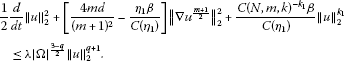Choosing ${\eta }_{1}$ small enough so that $\frac{4md}{{\left(m+1\right)}^{2}}-\frac{{\eta }_{1}\beta }{C\left({\eta }_{1}\right)}\ge 0$, we obtain the inequality

$\frac{d}{dt}{\parallel u\parallel }_{2}+{\parallel u\parallel }_{2}^{{k}_{1}-1}\left[\frac{C{\left(N,m,k\right)}^{-{k}_{1}}\beta }{C\left({\eta }_{1}\right)}-\lambda {|\mathrm{\Omega }|}^{\frac{3-q}{2}}{\parallel u\parallel }_{2}^{q-{k}_{1}+1}\right]\le 0.$

Hence, we have the inequality

$\frac{d}{dt}{\parallel u\parallel }_{2}+{C}_{3}{\parallel u\parallel }_{2}^{{k}_{1}-1}\le 0$

provided that

${\parallel {u}_{0}\parallel }_{2}<{\left[\frac{C{\left(N,m,k\right)}^{-{k}_{1}}\beta }{C\left({\eta }_{1}\right)\lambda {|\mathrm{\Omega }|}^{\frac{3-q}{2}}}\right]}^{\frac{1}{q-{k}_{1}+1}},$

and

$q>{k}_{1}-1=\frac{4k+N\left(m-k\right)}{4+N\left(m-k\right)},$

where ${C}_{3}=\frac{C{\left(N,m,k\right)}^{-{k}_{1}}\beta }{C\left({\eta }_{1}\right)}-\lambda {|\mathrm{\Omega }|}^{\frac{3-q}{2}}{\parallel {u}_{0}\parallel }_{2}^{q-{k}_{1}+1}>0$, from which and a similar argument as the one used in the proof of Theorem 1, the following decay estimates can be obtained:

$\begin{array}{c}{\parallel u\parallel }_{2}\le {\left[{\parallel {u}_{0}\parallel }_{2}^{2-{k}_{1}}-{C}_{3}\left(2-{k}_{1}\right)t\right]}^{\frac{1}{2-{k}_{1}}},\phantom{\rule{1em}{0ex}}t\in \left[0,{T}_{3}\right),\hfill \\ {\parallel u\parallel }_{2}=0,\phantom{\rule{1em}{0ex}}t\in \left[{T}_{3},+\mathrm{\infty }\right),\hfill \end{array}$

where ${T}_{3}=\frac{{\parallel {u}_{0}\parallel }_{2}^{2-{k}_{1}}}{{C}_{3}\left(2-{k}_{1}\right)}$.

If $N>2$ and $0, multiplying both sides of (1) by ${u}^{s}$ ($s>m$) and integrating the result over Ω, we get the equation

$\frac{1}{s+1}\frac{d}{dt}{\parallel u\parallel }_{s+1}^{s+1}+\frac{4msd}{{\left(m+s\right)}^{2}}{\parallel \mathrm{\nabla }{u}^{\frac{m+s}{2}}\parallel }_{2}^{2}+\beta {\parallel u\parallel }_{k+s}^{k+s}=\lambda {\int }_{\mathrm{\Omega }}{u}^{q}\phantom{\rule{0.2em}{0ex}}dx{\int }_{\mathrm{\Omega }}{u}^{s}\phantom{\rule{0.2em}{0ex}}dx.$
(19)

By (17) and (19), and using Hölder’s inequality, we obtain the inequality

$\begin{array}{c}\frac{1}{s+1}\frac{d}{dt}{\parallel u\parallel }_{s+1}^{s+1}+\left[\frac{4msd}{{\left(m+s\right)}^{2}}-\frac{{\eta }_{2}\beta }{C\left({\eta }_{2}\right)}\right]{\parallel \mathrm{\nabla }{u}^{\frac{m+s}{2}}\parallel }_{2}^{2}\hfill \\ \phantom{\rule{1em}{0ex}}+\frac{C{\left(N,k,s\right)}^{-{k}_{2}}\beta }{C\left({\eta }_{2}\right)}{\parallel u\parallel }_{s+1}^{{k}_{2}}\le \lambda {|\mathrm{\Omega }|}^{\frac{s-q+2}{s+1}}{\parallel u\parallel }_{s+1}^{q+s}.\hfill \end{array}$

Choosing ${\eta }_{2}$ small enough so that $\frac{4msd}{{\left(m+s\right)}^{2}}-\frac{{\eta }_{2}\beta }{C\left({\eta }_{2}\right)}\ge 0$, we have

$\frac{d}{dt}{\parallel u\parallel }_{s+1}+{\parallel u\parallel }_{s+1}^{{k}_{2}-s}\left[\frac{C{\left(N,k,s\right)}^{-{k}_{2}}\beta }{C\left({\eta }_{2}\right)}-\lambda {|\mathrm{\Omega }|}^{\frac{s-q+2}{s+1}}{\parallel u\parallel }_{s+1}^{q-{k}_{2}+s}\right]\le 0.$

Therefore, we obtain the inequality

$\frac{d}{dt}{\parallel u\parallel }_{s+1}+{C}_{4}{\parallel u\parallel }_{s+1}^{{k}_{2}-s}\le 0$

provided that

${\parallel {u}_{0}\parallel }_{s+1}<{\left[\frac{C{\left(N,k,s\right)}^{-{k}_{2}}\beta }{C\left({\eta }_{2}\right)\lambda {|\mathrm{\Omega }|}^{\frac{s-q+2}{s+1}}}\right]}^{\frac{1}{q-{k}_{2}+s}},$

and

$q>{k}_{2}-s=\frac{2k\left(s+1\right)+N\left(m-k\right)}{2\left(s+1\right)+N\left(m-k\right)},$

where ${C}_{4}=\frac{C{\left(N,k,s\right)}^{-{k}_{2}}\beta }{C\left({\eta }_{2}\right)}-\lambda {|\mathrm{\Omega }|}^{\frac{s-q+2}{s+1}}{\parallel {u}_{0}\parallel }_{s+1}^{q-{k}_{2}+s}>0$, which yields the following decay estimates:

$\begin{array}{c}{\parallel u\parallel }_{s+1}\le {\left[{\parallel {u}_{0}\parallel }_{s+1}^{s+1-{k}_{2}}-{C}_{4}\left(s+1-{k}_{2}\right)t\right]}^{\frac{1}{s+1-{k}_{2}}},\phantom{\rule{1em}{0ex}}t\in \left[0,{T}_{4}\right),\hfill \\ {\parallel u\parallel }_{s+1}=0,\phantom{\rule{1em}{0ex}}t\in \left[{T}_{4},+\mathrm{\infty }\right),\hfill \end{array}$

where ${T}_{4}=\frac{{\parallel {u}_{0}\parallel }_{s+1}^{s+1-{k}_{2}}}{{C}_{4}\left(s+1-{k}_{2}\right)}$.

Since $s>m$, we have $2\left(s+1\right)>2m+2$, and hence, if $k\ge m$, then $q>{k}_{2}-s\ge m$.

Assume that $q>1$. If ${\lambda }_{1}$ is the first eigenvalue of the boundary problem

$-\mathrm{\Delta }\psi \left(x\right)=\lambda \psi \left(x\right),\phantom{\rule{1em}{0ex}}x\in \mathrm{\Omega };\phantom{\rule{2em}{0ex}}\psi \left(x\right)=0,\phantom{\rule{1em}{0ex}}x\in \partial \mathrm{\Omega },$

and ${\phi }_{1}\left(x\right)\ge 0$, ${\parallel {\phi }_{1}\parallel }_{\mathrm{\infty }}=1$, is an eigenfunction corresponding to the eigenvalue ${\lambda }_{1}$, then for sufficiently small $a>0$, it can be easily shown that $a{\phi }_{1}^{\frac{1}{m}}\left(x\right)$ is an upper solution of problem (1)-(3) provided that ${u}_{0}\left(x\right)\le a{\phi }_{1}^{\frac{1}{m}}\left(x\right)$, $x\in \mathrm{\Omega }$. We then have $u\left(x,t\right)\le a{\phi }_{1}\left(x\right)$ for $t>0$ by the comparison principle. Therefore, from equation (19), we can obtain the inequality

$\begin{array}{c}\frac{1}{s+1}\frac{d}{dt}{\parallel u\parallel }_{s+1}^{s+1}+\left[\frac{4msd}{{\left(m+s\right)}^{2}}-\lambda {a}^{q-m}{C}_{0}^{2}{|\mathrm{\Omega }|}^{1+\frac{2}{N}}-\frac{{\eta }_{2}\beta }{C\left({\eta }_{2}\right)}\right]{\parallel \mathrm{\nabla }{u}^{\frac{m+s}{2}}\parallel }_{2}^{2}\hfill \\ \phantom{\rule{1em}{0ex}}+\frac{C{\left(N,k,s\right)}^{-{k}_{2}}\beta }{C\left({\eta }_{2}\right)}{\parallel u\parallel }_{s+1}^{{k}_{2}}\le 0,\hfill \end{array}$

from which the following decay estimates can be obtained:

$\begin{array}{c}{\parallel u\parallel }_{s+1}\le {\left[{\parallel {u}_{0}\parallel }_{s+1}^{s+1-{k}_{2}}-\frac{C{\left(N,k,s\right)}^{-{k}_{2}}\beta }{C\left({\eta }_{2}}\left(s+1-{k}_{2}\right)t\right]}^{\frac{1}{s+1-{k}_{2}}},\phantom{\rule{1em}{0ex}}t\in \left(0,{T}_{4}^{\ast }\right),\hfill \\ {\parallel u\parallel }_{s+1}=0,\phantom{\rule{1em}{0ex}}t\in \left[{T}_{4}^{\ast },+\mathrm{\infty }\right),\hfill \end{array}$

provided that

$\frac{4msd}{{\left(m+s\right)}^{2}}-\lambda {a}^{q-m}{C}_{0}^{2}{|\mathrm{\Omega }|}^{1+\frac{2}{N}}-\frac{{\eta }_{2}\beta }{C\left({\eta }_{2}\right)}\ge 0,$

where ${T}_{4}^{\ast }=\frac{C\left({\eta }_{2}\right){\parallel {u}_{0}\parallel }_{s+1}^{s+1-{k}_{2}}}{C{\left(N,k,s\right)}^{-{k}_{2}}\beta \left(s+1-{k}_{2}\right)}$. □

Remark 2 Since the Sobolev embedding inequality cannot be used in the proof of Theorem 2, it is not necessary to consider the cases that $\frac{N-2}{N+2}\le m<1$ and $0, when $N>2$. In addition, if $k\ge m$, the conditions in Theorem 2 imply that $q>m$.

Theorem 3 Suppose that $0 and $m>q\ge k$. Then the nonnegative nontrivial weak solution of problem (1)-(3) vanishes in finite time for any nonnegative initial data provided that β is sufficiently large.

Proof We first consider the case that . Multiplying both sides of (1) by u and integrating the result over Ω, and using Hölder’s inequality, we get

$\begin{array}{c}\frac{1}{2}\frac{d}{dt}{\parallel u\parallel }_{2}^{2}+\frac{4md}{{\left(m+1\right)}^{2}}{\parallel \mathrm{\nabla }{u}^{\frac{m+1}{2}}\parallel }_{2}^{2}+\beta {\parallel u\parallel }_{k+1}^{k+1}\hfill \\ \phantom{\rule{1em}{0ex}}=\lambda {\int }_{\mathrm{\Omega }}{u}^{q}\phantom{\rule{0.2em}{0ex}}dx{\int }_{\mathrm{\Omega }}u\phantom{\rule{0.2em}{0ex}}dx\le \lambda |\mathrm{\Omega }|{\parallel u\parallel }_{q+1}^{q+1}.\hfill \end{array}$

By Lemma 2, we have the inequality

${\parallel u\parallel }_{q+1}\le C\left(N,k,q\right){\parallel u\parallel }_{k+1}^{1-{\theta }_{3}}{\parallel \mathrm{\nabla }{u}^{\frac{m+1}{2}}\parallel }_{2}^{\frac{2{\theta }_{3}}{m+1}},$
(20)

where ${\theta }_{3}=\frac{m+1}{2}\left(\frac{1}{k+1}-\frac{1}{q+1}\right){\left(\frac{1}{N}-\frac{1}{2}+\frac{m+1}{2}\frac{1}{k+1}\right)}^{-1}=\frac{N\left(q-k\right)\left(m+1\right)}{\left(q+1\right)\left[2\left(k+1\right)+N\left(m-k\right)\right]}\in \left[0,1\right)$. Since $q, we have $m+1-\left(q+1\right){\theta }_{3}>0$. It then follows from (20) and Young’s inequality that

$\begin{array}{rcl}\lambda |\mathrm{\Omega }|{\parallel u\parallel }_{q+1}^{q+1}& \le & \lambda |\mathrm{\Omega }|C{\left(N,k,q\right)}^{q+1}{\parallel u\parallel }_{k+1}^{\left(q+1\right)\left(1-{\theta }_{3}\right)}{\parallel \mathrm{\nabla }{u}^{\frac{m+1}{2}}\parallel }_{2}^{\frac{2\left(q+1\right){\theta }_{3}}{m+1}}\\ \le & \lambda |\mathrm{\Omega }|C{\left(N,k,q\right)}^{q+1}\left({\eta }_{3}{\parallel \mathrm{\nabla }{u}^{\frac{m+1}{2}}\parallel }_{2}^{2}+C\left({\eta }_{3}\right){\parallel u\parallel }_{k+1}^{\frac{\left(q+1\right)\left(1-{\theta }_{3}\right)\left(m+1\right)}{m+1-\left(q+1\right){\theta }_{3}}}\right),\end{array}$
(21)

where ${\eta }_{3}$ will be determined later. From (18) and (21), one can see that

$\begin{array}{c}\frac{1}{2}\frac{d}{dt}{\parallel u\parallel }_{2}^{2}+\left[\frac{4md}{{\left(m+1\right)}^{2}}-{\eta }_{3}\lambda |\mathrm{\Omega }|C{\left(N,k,q\right)}^{q+1}\right]{\parallel \mathrm{\nabla }{u}^{\frac{m+1}{2}}\parallel }_{2}^{2}+\beta {\parallel u\parallel }_{k+1}^{k+1}\hfill \\ \phantom{\rule{1em}{0ex}}\le C\left({\eta }_{3}\right)\lambda |\mathrm{\Omega }|C{\left(N,k,q\right)}^{q+1}{\parallel u\parallel }_{k+1}^{\frac{\left(q+1\right)\left(1-{\theta }_{3}\right)\left(m+1\right)}{m+1-\left(q+1\right){\theta }_{3}}}.\hfill \end{array}$

We then obtain the inequality

$\begin{array}{c}\frac{1}{2}\frac{d}{dt}{\parallel u\parallel }_{2}^{2}+\left[\frac{4md}{{\left(m+1\right)}^{2}}-{\eta }_{3}\lambda |\mathrm{\Omega }|C{\left(N,k,q\right)}^{q+1}\right]{\gamma }^{-2}{\parallel u\parallel }_{2}^{m+1}\hfill \\ \phantom{\rule{1em}{0ex}}+{\parallel u\parallel }_{k+1}^{k+1}\left[\beta -C\left({\eta }_{3}\right)\lambda |\mathrm{\Omega }|C{\left(N,k,q\right)}^{q+1}{\parallel u\parallel }_{k+1}^{{\alpha }_{1}}\right]\le 0\hfill \end{array}$

by (6) and the inequality above, where ${\alpha }_{1}=\frac{\left(q+1\right)\left(1-{\theta }_{3}\right)\left(m+1\right)}{m+1-\left(q+1\right){\theta }_{3}}-\left(k+1\right)=\frac{2\left(q-k\right)\left(k+1\right)}{2\left(k+1\right)+N\left(m-q\right)}\ge 0$. We can choose ${\eta }_{3}$ small enough so that ${C}_{5}=\frac{4md}{{\left(m+1\right)}^{2}}-{\eta }_{3}\lambda |\mathrm{\Omega }|C{\left(N,k,q\right)}^{q+1}>0$. Once ${\eta }_{3}$ is fixed, we may choose β large enough so that

$\beta -C\left({\eta }_{3}\right)\lambda |\mathrm{\Omega }|C{\left(N,k,q\right)}^{q+1}{\parallel u\parallel }_{k+1}^{{\alpha }_{1}}\ge 0.$

Hence, we have the inequality

$\frac{d}{dt}{\parallel u\parallel }_{2}+{C}_{5}{\parallel u\parallel }_{2}^{m}\le 0,$

from which the following decay estimates can be obtained by a similar argument as the one used in the proof of Theorem 1:

$\begin{array}{c}{\parallel u\parallel }_{2}\le {\left[{\parallel {u}_{0}\parallel }_{2}^{1-m}-{C}_{5}\left(1-m\right)t\right]}^{\frac{1}{1-m}},\phantom{\rule{1em}{0ex}}t\in \left[0,{T}_{5}\right),\hfill \\ {\parallel u\parallel }_{2}=0,\phantom{\rule{1em}{0ex}}t\in \left[{T}_{5},+\mathrm{\infty }\right),\hfill \end{array}$

where ${T}_{5}=\frac{{\parallel {u}_{0}\parallel }_{2}^{1-m}}{{C}_{5}\left(1-m\right)}$.

Secondly, we consider the case that $N>2$. If $\frac{N-2}{N+2}\le m<1$, multiplying both sides of (1) by ${u}^{s}$ ($s>m$) and integrating the result over Ω, and then using Hölder’s inequality, we get

$\frac{1}{s+1}\frac{d}{dt}{\parallel u\parallel }_{s+1}^{s+1}+\frac{4msd}{{\left(m+s\right)}^{2}}{\parallel \mathrm{\nabla }{u}^{\frac{m+s}{2}}\parallel }_{2}^{2}+\beta {\parallel u\parallel }_{k+s}^{k+s}=\lambda {\int }_{\mathrm{\Omega }}{u}^{q}\phantom{\rule{0.2em}{0ex}}dx{\int }_{\mathrm{\Omega }}{u}^{s}\phantom{\rule{0.2em}{0ex}}dx\le \lambda |\mathrm{\Omega }|{\parallel u\parallel }_{q+s}^{q+s}.$

By Lemma 2, it can be shown that

${\parallel u\parallel }_{q+s}\le C\left(N,k,q,s\right){\parallel u\parallel }_{k+s}^{1-{\theta }_{4}}{\parallel \mathrm{\nabla }{u}^{\frac{m+s}{2}}\parallel }_{2}^{\frac{2{\theta }_{4}}{m+s}},$
(22)

where ${\theta }_{4}=\frac{m+s}{2}\left(\frac{1}{k+s}-\frac{1}{q+s}\right){\left(\frac{1}{N}-\frac{1}{2}+\frac{m+s}{2}\frac{1}{k+s}\right)}^{-1}=\frac{N\left(q-k\right)\left(m+s\right)}{\left(q+s\right)\left[2\left(k+s\right)+N\left(m-k\right)\right]}\in \left[0,1\right)$. Since $q, we have $m+s-\left(q+s\right){\theta }_{4}>0$. It then follows from (22) and Young’s inequality that

$\begin{array}{rcl}\lambda |\mathrm{\Omega }|{\parallel u\parallel }_{q+s}^{q+s}& \le & \lambda |\mathrm{\Omega }|C{\left(N,k,q,s\right)}^{q+s}{\parallel u\parallel }_{k+s}^{\left(q+s\right)\left(1-{\theta }_{4}\right)}{\parallel \mathrm{\nabla }{u}^{\frac{m+s}{2}}\parallel }_{2}^{\frac{2\left(q+s\right){\theta }_{4}}{m+s}}\\ \le & \lambda |\mathrm{\Omega }|C{\left(N,k,q,s\right)}^{q+s}\left({\eta }_{4}{\parallel \mathrm{\nabla }{u}^{\frac{m+s}{2}}\parallel }_{2}^{2}+C\left({\eta }_{4}\right){\parallel u\parallel }_{k+s}^{\frac{\left(q+s\right)\left(1-{\theta }_{4}\right)\left(m+s}{m+s-\left(q+s\right){\theta }_{4}}}\right),\end{array}$
(23)

where ${\eta }_{4}$ will be determined later. From (19) and (23), one can see that

$\begin{array}{c}\frac{1}{s+1}\frac{d}{dt}{\parallel u\parallel }_{s+1}^{s+1}+\left[\frac{4msd}{{\left(m+s\right)}^{2}}-{\eta }_{4}\lambda |\mathrm{\Omega }|C{\left(N,k,q,s\right)}^{q+s}\right]{\parallel \mathrm{\nabla }{u}^{\frac{m+s}{2}}\parallel }_{2}^{2}+\beta {\parallel u\parallel }_{k+s}^{k+s}\hfill \\ \phantom{\rule{1em}{0ex}}\le C\left({\eta }_{4}\right)\lambda |\mathrm{\Omega }|C{\left(N,k,q,s\right)}^{q+s}{\parallel u\parallel }_{k+s}^{\frac{\left(q+s\right)\left(1-{\theta }_{4}\right)\left(m+s\right)}{m+s-\left(q+s\right){\theta }_{4}}}.\hfill \end{array}$

By (12), (13), and the inequality above, we can obtain the inequality

$\begin{array}{c}\frac{1}{s+1}\frac{d}{dt}{\parallel u\parallel }_{s+1}^{s+1}+\left[\frac{4msd}{{\left(m+s\right)}^{2}}-{\eta }_{4}\lambda |\mathrm{\Omega }|C{\left(N,k,q,s\right)}^{q+s}\right]{C}_{0}^{-2}{|\mathrm{\Omega }|}^{\frac{N-2}{N}-\frac{m+s}{s+1}}{\parallel u\parallel }_{s+1}^{m+s}\hfill \\ \phantom{\rule{1em}{0ex}}+{\parallel u\parallel }_{k+s}^{k+s}\left[\beta -C\left({\eta }_{4}\right)\lambda |\mathrm{\Omega }|C{\left(N,k,q,s\right)}^{q+s}{\parallel u\parallel }_{k+s}^{{\alpha }_{2}}\right]\le 0,\hfill \end{array}$

where ${\alpha }_{2}=\frac{\left(q+s\right)\left(1-{\theta }_{4}\right)\left(m+s\right)}{m+s-\left(q+s\right){\theta }_{4}}-\left(k+s\right)=\frac{2\left(q-k\right)\left(k+s\right)}{2\left(k+s\right)+N\left(m-q\right)}\ge 0$. We can choose ${\eta }_{4}$ small enough so that ${C}_{6}=\left[\frac{4msd}{{\left(m+s\right)}^{2}}-{\eta }_{4}\lambda |\mathrm{\Omega }|C{\left(N,k,q,s\right)}^{q+s}\right]{C}_{0}^{-2}{|\mathrm{\Omega }|}^{\frac{N-2}{N}-\frac{m+s}{s+1}}>0$. Once ${\eta }_{4}$ is fixed, we can choose β large enough so that

$\beta -C\left({\eta }_{4}\right)\lambda |\mathrm{\Omega }|C{\left(N,k,q,s\right)}^{q+s}{\parallel u\parallel }_{k+s}^{{\alpha }_{2}}\ge 0.$

Hence, we can obtain the inequality

$\frac{d}{dt}{\parallel u\parallel }_{s+1}+{C}_{6}{\parallel u\parallel }_{s+1}^{m}\le 0,$

from which the following decay estimates can be obtained:

$\begin{array}{c}{\parallel u\parallel }_{s+1}\le {\left[{\parallel {u}_{0}\parallel }_{s+1}^{1-m}-{C}_{6}\left(1-m\right)t\right]}^{\frac{1}{1-m}},\phantom{\rule{1em}{0ex}}t\in \left[0,{T}_{6}\right),\hfill \\ {\parallel u\parallel }_{s+1}=0,\phantom{\rule{1em}{0ex}}t\in \left[{T}_{6},+\mathrm{\infty }\right),\hfill \end{array}$

where ${T}_{6}=\frac{{\parallel {u}_{0}\parallel }_{s+1}^{1-m}}{{C}_{6}\left(1-m\right)}$.

Similarly, one can obtain the following decay estimates for $0: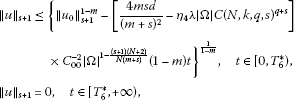where ${T}_{6}^{\ast }=\frac{{\parallel {u}_{0}\parallel }_{s+1}^{1-m}}{\left[\frac{4msd}{{\left(m+s\right)}^{2}}-{\eta }_{4}\lambda |\mathrm{\Omega }|C{\left(N,k,q,s\right)}^{q+s}\right]{C}_{00}^{-2}{|\mathrm{\Omega }|}^{1-\frac{\left(s+1\right)\left(N+2\right)}{N\left(m+s\right)}}\left(1-m\right)}$. □

Remark 3 One can see from Theorems 1-3 that the extinction of nonnegative nontrivial weak solutions to problem (1)-(3) occurs when $0.

Remark 4 Theorems 1-3 all require $|\mathrm{\Omega }|$, λ, or ${u}_{0}$ to be sufficiently small or β to be sufficiently large.

## References

1. Bebernes J, Eberly D: Mathematical Problems from Combustion Theory. Springer, New York; 1989.

2. Pao CV: Nonlinear Parabolic and Elliptic Equations. Plenum, New York; 1992.

3. Furter J, Grinfield M: Local vs. nonlocal interactions in populations dynamics. J. Math. Biol. 1989, 27: 65-80. 10.1007/BF00276081

4. Chen YP: Blow-up for a system of heat equations with nonlocal sources and absorptions. Comput. Math. Appl. 2004, 48: 361-372. 10.1016/j.camwa.2004.05.002

5. Souplet P: Blow-up in nonlocal reaction-diffusion equations. SIAM J. Math. Anal. 1998, 29: 1301-1334. 10.1137/S0036141097318900

6. Souplet P: Uniform blow-up profiles and boundary behavior for diffusion equations with nonlocal nonlinear source. J. Differ. Equ. 1999, 153: 374-406. 10.1006/jdeq.1998.3535

7. Wang MX, Wang YM: Properties of positive solutions for non-local reaction-diffusion problems. Math. Methods Appl. Sci. 1996, 19: 1141-1156. 10.1002/(SICI)1099-1476(19960925)19:14<1141::AID-MMA811>3.0.CO;2-9

8. Galaktionov VA, Levine HA: A general approach to critical Fujita exponents in nonlinear parabolic problems. Nonlinear Anal. 1998, 34: 1005-1027. 10.1016/S0362-546X(97)00716-5

9. Afanas’eva NV, Tedeev AF: Theorems on the existence and nonexistence of solutions of the Cauchy problem for degenerate parabolic equations with nonlocal source. Ukr. Math. J. 2005, 57: 1687-1711. 10.1007/s11253-006-0024-6

10. Evans LC, Knerr BF: Instantaneous shrinking of the support of nonnegative solutions to certain nonlinear parabolic equations and variational inequalities. Ill. J. Math. 1979, 23: 153-166.

11. Ferreira R, Vazquez JL: Extinction behavior for fast diffusion equations with absorption. Nonlinear Anal. 2001, 43: 353-376.

12. Li YX, Wu JC: Extinction for fast diffusion equations with nonlinear sources. Electron. J. Differ. Equ. 2005., 2005: Article ID 23

13. Yin JX, Jin CH: Critical extinction and blow-up exponents for fast diffusive Laplacian with sources. Math. Methods Appl. Sci. 2007, 30: 1147-1167. 10.1002/mma.833

14. Zhou J, Mu CL: Critical blow-up and extinction exponents for non-Newton polytropic filtration equation with source. Bull. Korean Math. Soc. 2009, 46: 1159-1173. 10.4134/BKMS.2009.46.6.1159

15. Liu WJ, Wang MX, Wu B: Extinction and decay estimates of solutions for a class of porous medium equations. J. Inequal. Appl. 2007., 2007: Article ID 087650

16. Fang ZB, Li G: Extinction and decay estimates of solutions for a class of doubly degenerate equations. Appl. Math. Lett. 2012, 25: 1795-1802. 10.1016/j.aml.2012.02.020

17. Han YZ, Gao WJ: Extinction for a fast diffusion equation with a nonlinear nonlocal source. Arch. Math. 2011, 97: 353-363. 10.1007/s00013-011-0299-1

18. Liu WJ: Extinction and non-extinction of solutions for a nonlocal reaction-diffusion problem. Electron. J. Qual. Theory Differ. Equ. 2010., 2010: Article ID 15

19. Fang ZB, Xu XH: Extinction behavior of solutions for the p -Laplacian equations with nonlocal sources. Nonlinear Anal., Real World Appl. 2012, 13: 1780-1789. 10.1016/j.nonrwa.2011.12.008

20. Antontsev S, Diaz JI, Shmarev S Progress in Nonlinear Differential Equations and Their Applications. In Energy Methods for Free Boundary Problems: Applications to Nonlinear PDEs and Fluid Mechanics. Birkhäuser, Boston; 2002.

21. Sacks PE: Continuity of solutions of a singular parabolic equation. Nonlinear Anal. 1983, 7: 387-409. 10.1016/0362-546X(83)90092-5

22. Wu ZQ, Zhao JN, Yin JX, Li HL: Nonlinear Diffusion Equations. World Scientific, River Edge; 2001.

23. Ladyzhenskaya OA, Solonnikov VA, Ural’tseva NN: Linear and Quasilinear Equations of Parabolic Type. Am. Math. Soc., Providence; 1968.

## Acknowledgements

The second and third authors were supported by the National Science Foundation of Shandong Province of China (ZR2012AM018) and Changwon National University in 2012, respectively. The authors would like to express their sincere gratitude to the anonymous reviewers for their insightful and constructive comments.

## Author information

Authors

### Corresponding author

Correspondence to Zhong Bo Fang.

### Competing interests

The authors declare that they have no competing interests.

### Authors’ contributions

All authors contributed equally to the manuscript and read and approved the final manuscript.

## Rights and permissions

Reprints and Permissions

Xu, X., Fang, Z.B. & Yi, SC. Extinction and decay estimates of solutions for a porous medium equation with nonlocal source and strong absorption. Bound Value Probl 2013, 24 (2013). https://doi.org/10.1186/1687-2770-2013-24

• Accepted:

• Published:

• DOI: https://doi.org/10.1186/1687-2770-2013-24

### Keywords

• Decay Estimate
• Nonlinear Parabolic Equation
• Porous Medium Equation
• Nonlocal Term
• Extinction Behavior